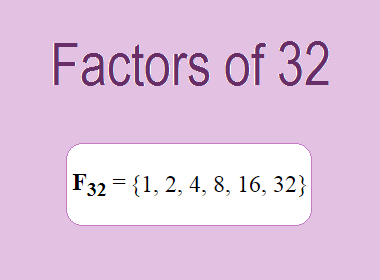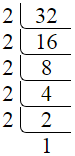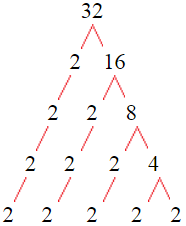# Factors of 32The factors of 32 are 1, 2, 4, 8, 16, and 32 i.e. F32 = {1, 2, 4, 8, 16, 32}. The factors of 32 are all the numbers that can divide 32 without leaving a remainder.

We can check if these numbers are factors of 32 by dividing 32 by each of them. If the result is a whole number, then the number is a factor of 32. Let's do this for each of the numbers listed above:

·        1 is a factor of 32 because 32 divided by 1 is 32.

·        2 is a factor of 32 because 32 divided by 2 is 16.

·        4 is a factor of 32 because 32 divided by 4 is 8.

·        8 is a factor of 32 because 32 divided by 8 is 4.

·        16 is a factor of 32 because 32 divided by 16 is 2.

·        32 is a factor of 32 because 32 divided by 32 is 1.

## How to Find Factors of 32?

1 and the number itself are the factors of every number. So, 1 and 32 are two factors of 32. To find the other factors of 32, we can start by dividing 32 by the numbers between 1 and 32. If we divide 32 by 2, we get a remainder of 0. Therefore, 2 is a factor of 32. If we divide 32 by 3, we get a remainder of 2. Therefore, 3 is not a factor of 32.

Next, we can check if 4 is a factor of 32. If we divide 32 by 4, we get a remainder of 0. Therefore, 4 is also a factor of 32. We can continue this process for all the possible factors of 32.

Through this process, we can find that the factors of 32 are 1, 2, 4, 8, 16, and 32. These are the only numbers that can divide 32 without leaving a remainder.

********************

********************

## Properties of the Factors of 32

The factors of 32 have some interesting properties. One of the properties is that the sum of the factors of 32 is equal to 63. We can see this by adding all the factors of 32 together:

1 + 2 + 4 + 8 + 16 + 32 = 63

Another property of the factors of 32 is that the prime factor of 32 is 2 only.

## Applications of the Factors of 32

The factors of 32 have several applications in mathematics. One of the applications is in finding the highest common factor (HCF) of two or more numbers. The HCF is the largest factor that two or more numbers have in common. For example, to find the HCF of 32 and 40, we need to find the factors of both numbers and identify the largest factor they have in common. The factors of 32 are 1, 2, 4, 8, 16, and 32. The factors of 40 are 1, 2, 4, 5, 8, 10, 20, and 40. The largest factor that they have in common is 8. Therefore, the HCF of 32 and 40 is 8.

Another application of the factors of 32 is in prime factorization. Prime factorization is the process of expressing a number as the product of its prime factors. The only prime factor of 32 is 2. Therefore, we can express 32 as:

32 = 2 × 2 × 2 × 2 × 2

We can do prime factorization by division and factor tree method also. Here is the prime factorization of 32 by division method,32 = 2 × 2 × 2 × 2 × 2

Here is the prime factorization of 32 by the factor tree method,32 = 2 × 2 × 2 × 2 × 2

## Conclusion

The factors of 32 are the numbers that can divide 32 without leaving a remainder. The factors of 32 are 1, 2, 4, 8, 16, and 32. The factors of 32 have some interesting properties, such as having a sum of 63. The factors of 32 have several applications in mathematics, such as finding the highest common factor and prime factorization.